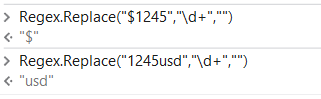# Want help to build a regex expression

how to write a regex expression where the expression checks whether a number is starting or ending with a set list of characters…like USD,eur,ron,INR,\$,€ for example \$1245 should return \$ as a result and 1245usd should return usd as a result

HI,

``````System.Text.RegularExpressions.Regex.Match(yourString,"\p{Sc}(?=\d+)|(?<=\d+)[A-Za-z]{3}\b").Value
``````

Regards,

1 Like

Hello @Debojyoti_chanda
Try this Expression
Var1=“\$500”

``````System.Text.RegularExpressions.Regex.Match(Var1,"\W(?=\d)|\D+(?=\d)").Tostring.trim
``````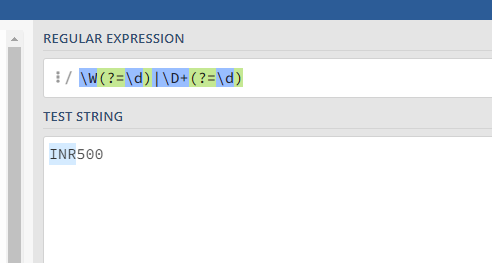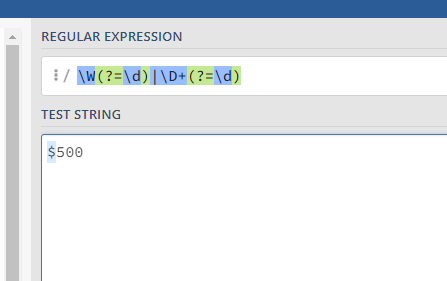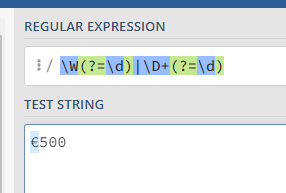when regulating more specific then keep in mind that certain amount of currency codes / chars are to handle. Maybe some generics for a subset will serve your case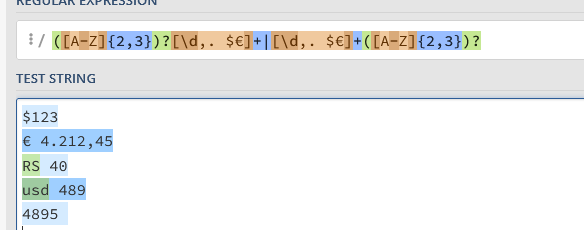`([A-Z]{2,3})?[\d,. \$€]+|[\d,. \$€]+([A-Z]{2,3})?`
used along with the case insensitive option

We would special highlight from @Yoichi ’ suggestion the `\p{Sc}` representing currency symbols
which we combine to [\d,. \p{Sc}]

we combined amount and currency for a better match with generics (e.g. 2 or 3 letter codes) as we want to avoid to match any 2 or 3 letters and forcing that there are numbers in the neighborhood.

With grouping the 2 components we can later retrieve only the currency info

This works fine if there is something in front like \$12345 but doesn’t work for something like this 78787usd or 12345\$

@Debojyoti_chanda , Try this

``````\W(?=\d)|\D+(?=\d)|(?!\d+)\D+
``````
``````System.Text.RegularExpressions.Regex.Match(Var1,"\W(?=\d)|\D+(?=\d)|(?!\d+)\D+").Tostring.trim
``````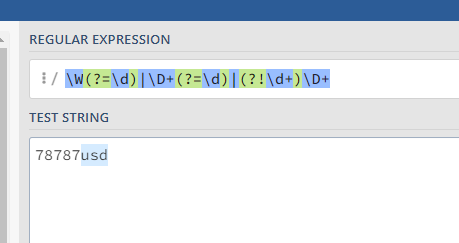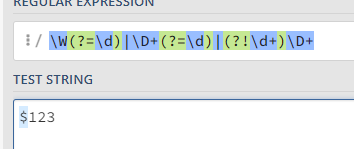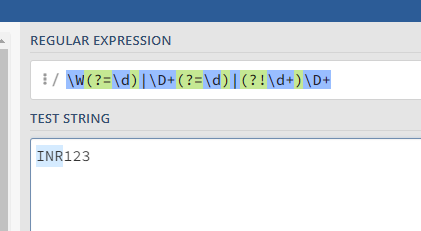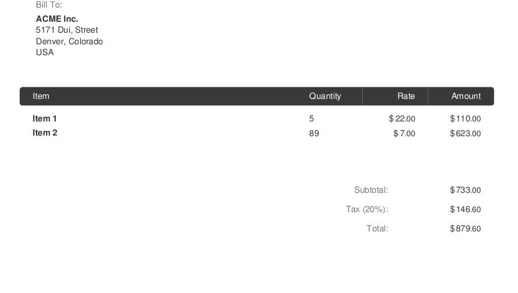actually i am using regex based document understanding and i have invoices like these two and want regex based extractor to extarct the currency

((?:usd))((?:ron))((?:eur))((?:inr))((?:\$))((?:€))((?:₹))\d\$|^\d*\s((?:usd))((?:ron))((?:eur))((?:inr))((?:\$))((?:€))((?:₹)*)

this seems like working for me